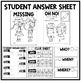# 6th Grade Math Test Prep Task Cards Solve the Mystery of the Missing PencilsSubject
Resource Type
File Type

PDF

(41 MB|62 pages)
Product Rating
Standards
• Product Description
• StandardsNEW

• Directions
• Who, When, and Where Posters (6 pages)
• Case of the Missing Pencils Letter (color and b & w)
• Posters (15 pages)
• 9 Task cards (color and b & w)
• Student Journals and answer sheet (3 pages)
• Clues (11 pages)
• Student Awards (color and b & w)

Content Covered

• Finding greatest common factors
• Solving one-step equations
• Plotting coordinate pairs
• Comparing integers and rational numbers
• Finding area, perimeter, surface area and volume
• The distributive property
• Writing equations from story problems
• Solving inequalities
• Percent problems
• Finding mean from a line plot
• Constructing histograms
• Finding range and upper quartiles from a table

I also have a paper/pencil test prep and a set of Google Classroom test prep task cards if you are interested.

Some fun ways to connect....

FOLLOW MY JOURNEY AT MY BLOG.

Understand the concept of a unit rate 𝘢/𝘣 associated with a ratio 𝘢:𝘣 with 𝘣 ≠ 0, and use rate language in the context of a ratio relationship. For example, “This recipe has a ratio of 3 cups of flour to 4 cups of sugar, so there is 3/4 cup of flour for each cup of sugar.” “We paid \$75 for 15 hamburgers, which is a rate of \$5 per hamburger.”
Understand the concept of a ratio and use ratio language to describe a ratio relationship between two quantities. For example, “The ratio of wings to beaks in the bird house at the zoo was 2:1, because for every 2 wings there was 1 beak.” “For every vote candidate A received, candidate C received nearly three votes.”
Write an inequality of the form 𝘹 > 𝘤 or 𝘹 < 𝘤 to represent a constraint or condition in a real-world or mathematical problem. Recognize that inequalities of the form 𝘹 > 𝘤 or 𝘹 < 𝘤 have infinitely many solutions; represent solutions of such inequalities on number line diagrams.
Solve real-world and mathematical problems by writing and solving equations of the form 𝘹 + 𝘱 = 𝘲 and 𝘱𝘹 = 𝘲 for cases in which 𝘱, 𝘲 and 𝘹 are all nonnegative rational numbers.
Use variables to represent numbers and write expressions when solving a real-world or mathematical problem; understand that a variable can represent an unknown number, or, depending on the purpose at hand, any number in a specified set.
Total Pages
62 pages
Included
Teaching Duration
N/A
Report this Resource to TpT
Reported resources will be reviewed by our team. Report this resource to let us know if this resource violates TpT’s content guidelines.